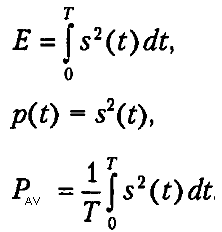# Average Speed and Average Power confusion

I found it somehow tricky to understand average speed and average power in mathematical fashion.
I suppose general idea of taking average of some quantity is: sum of all values/number of values.
how that idea fit's into idea of average speed=distance traveled/time traveled

similarly, average signal power:why is it over T?

A.T.
I found it somehow tricky to understand average speed and average power in mathematical fashion.
I suppose general idea of taking average of some quantity is: sum of all values/number of values.
how that idea fit's into idea of average speed=distance traveled/time traveled

similarly, average signal power:why is it over T?

Do you understand integrals in discrete terms?

https://betterexplained.com/articles/a-calculus-analogy-integrals-as-multiplication/

•LLT71
robphy
Homework Helper
Gold Member
time-weighted average of Q(t)=##\displaystyle\frac{\int_0^T Q(t)\ dt}{ \int_0^T \ dt }=\frac{\int_0^T Q(t)\ dt}{ T } ##
(For example, the average-velocity is a time-weighted average of velocity:
##\displaystyle\frac{\int_{t_1}^{t_2} v(t) \ dt}{ \int_{t_1}^{t_2} \ dt }=\frac{\int_{t_1}^{t_2} \frac{dx(t)}{dt} \ dt}{ \int_{t_1}^{t_2} \ dt }=\frac{x(t_2)-x(t_1)}{ t_2-t_1}=\frac{\Delta x}{ \Delta t} ##.

the average-speed is a time-weighted average of speed:
##\displaystyle\frac{\int_{t_1}^{t_2} |v(t)| \ dt}{ \int_{t_1}^{t_2} \ dt }=\frac{\int_{t_1}^{t_2} |\frac{dx(t)}{dt}| \ dt}{ \int_{t_1}^{t_2} \ dt }=\frac{d}{ t_2-t_1}=\frac{d}{ \Delta t} ##.
)

z-weighted average of Q(z)=##\displaystyle\frac{\int_0^Z Q(z)\ dz}{ \int_0^Z \ dz }=\frac{\int_0^Z Q(z)\ dz}{ Z }##
(For example, the center of mass is a mass-weighted average of position:
##\displaystyle\frac{m_1x_1+m_2x_2+m_3x_3 }{m_1+m_2+m_3}=\frac{m_1x_1+m_2x_2+m_3x_3 }{M}##.
If all of the "masses" are equal (so each item has equal "weight" in the average),
then the mass-weighted average reduces to
the count-weighted (ordinary, "straight") average:
##\displaystyle\frac{(1)x_1+(1)x_2+(1)x_3 }{(1)+(1)+(1)}=\frac{x_1+x_2+x_3 }{3}##.
)

•LLT71
thanks guys!